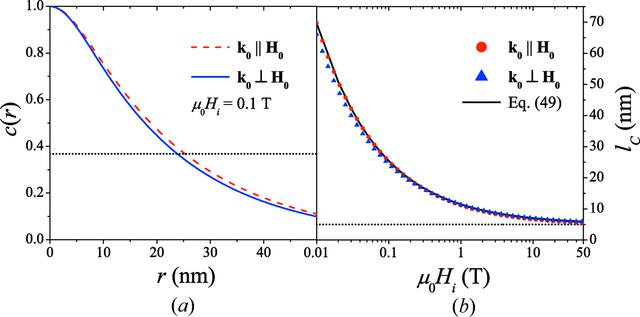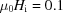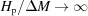disable zoom     view article Figure 5 (a) Comparison of the c(r) for the two scattering geometries [T; S(q) = 1;]. Dotted horizontal line: c(r) = exp(-1). (b) Comparison of the field dependence of the spin-misalignment correlation length lC for the two scattering geometries [S(q) = 1;] (log–linear scale). Solid line: equation (49). Dotted horizontal line: lC = R = 5 nm.JOURNAL OFAPPLIEDCRYSTALLOGRAPHY
ISSN: 1600-5767
Volume 48| Part 5| October 2015| Pages 1437-1450